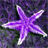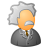# Find maximum value across a row

8471
5
04-29-2013 05:24 PMOccasional Contributor
Hi,

I am wondering if there is a tool that will look at a row, find the maximum value from column A, B, or C and then update column D with the name of the highest-ranked column. I could implement this as a cursor in Python but I would like to know if there is a set-based method that could be performed with ArcGIS Desktop (I have previously done this in SQL Server, but want to keep the requirements to a minimum).

At this stage the data is a polygon derived from an intersect, but it could also be modelled as a series of rasters.

Thanks,

Matt
Tags (3)
5 RepliesOccasional Contributor II
Hi,
I think that it could be quite easy to implement it in Field Calculator, unless there are to many fields to check. Never heard about out-of-box solution. I would write python script as well.

Regards
ArekFrequent Contributor II
If you only need to do it the one time then simply use a combination of Select by Attributes and Field Caluclator on the table.

Select by attributes

columnA > columnB and ColumnA > columnC

This will give you all the columnA which are larger then both B and C

Then from that selection query populate columnD with the Value of ColumnA

Then do similar queries for ColumnB and ColumnC

Do it three times and you are done.

You cold also create this as tool in model builder and save it as a button.

Out of the box.  But as I wrote if you only need to do it once or every few months it is just as well to do it with select by attributes and field calculator.

Hi,

I am wondering if there is a tool that will look at a row, find the maximum value from column A, B, or C and then update column D with the name of the highest-ranked column. I could implement this as a cursor in Python but I would like to know if there is a set-based method that could be performed with ArcGIS Desktop (I have previously done this in SQL Server, but want to keep the requirements to a minimum).

At this stage the data is a polygon derived from an intersect, but it could also be modelled as a series of rasters.

Thanks,

MattRegular Contributor
You can use Calculate Field.  The attached shows a graphic of a table named "A" with three numeric fields.  In the Expression, I call the getMaxField routine I wrote passing in the values of the three fields and the names of the three fields:

`getMaxField(!FIELD_A_1!, !FIELD_A_2!, !FIELD_A_3!, "FIELD_A_1", "FIELD_A_2", "FIELD_A_3" )`

The Code Block:
`def getMaxField(v1, v2, v3, name1, name2, name3):  maxval = max(v1,v2,v3)  if maxval == v1:    return name1  if maxval == v2:   return name2  return name3`Occasional Contributor
Thanks for your answers.

Dale's code block is similar to the SQL I have used for this problem. I must remember that the code block can be quite powerful!

One thing I didn't realise is that the number of columns will vary with each dataset this is run on - there could be up to six colums or only one. So defining the function to accommodate this could be tricky. I guess I could just define all six and add any missing columns so the expression doen't return an error.

I will try to implement this later and post back.

Thanks,

MattRegular Contributor
I played around with this a bit more given that you can't know the number of fields at runtime.
What you can do is receive the arguments as a tuple.  So, in the code block, you do this:
`def getMaxField(*args):`

All the arguments are now available to you.  Assuming that you'll pass in the field values followed by the field names like this:
`getMaxField(!FIELD_A_1!, !FIELD_A_2!, !FIELD_A_3!, "FIELD_A_1", "FIELD_A_2", "FIELD_A_3" )`

You can receive the arguments and split them up into two arrays, one of values, one of names.  To get the total number of args, use the len() function.  Here is the code to create two arrays:
`  nfields = len(args)  vals = args[0:nfields/2]  fields = args[nfields/2:nfields]`

Next, we need to find the maximum value in the vals array.  Python has the max() function:
`maxValue = max(vals)`

But we don't want just the max value, we want the position in the array of the max value.  Python lists/tuples have the ().index method.  So, to find the index of the max value:
`  i = vals.index(max(vals))`

Now all that's left is to return the name of the field found at that index:
`  return fields`

Here's the completed (verbose) code.  It worked for my simple test case.  It doesn't do anything about duplicate maximum values in the array.
`def getMaxField(*args):  nfields = len(args)  vals = args[0:nfields/2]  fields = args[nfields/2:nfields]  i = vals.index(max(vals))  return fields`